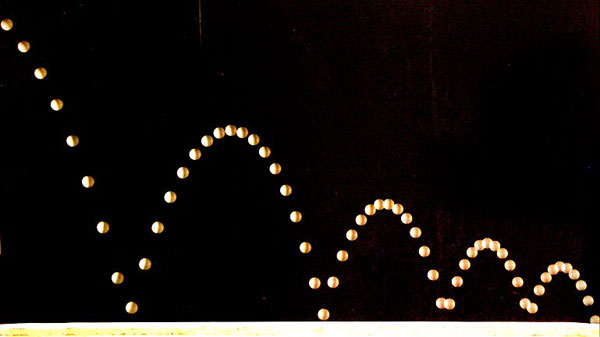SEARCH HOMEMath Central Quandaries & QueriesHello, Wondering if there is a way to figure out the quadratic equation of half of a parabola? Doing an assignment about finding quadratic equations for separate bounces of a ping pong ball, but the first bounce is incomplete (as the ball has presumably been dropped, hit the ground and then went on to make a complete bounce). Please see attachment for references to the diagram that I am using. Not sure whether I would have to look at it from a different perspective. Thanks, AbiHi Abi,Put a coordinate system on your diagram with the X-axis along the ping pong table and the Y-axis at the left of your diagram. Assume that the player serving the ball releases it $b$ centimeters above the table and at the same instant strikes it with the paddle moving in the positive X direction giving it an initial velocity in the X direction as $v_0$ centimeters per second. If you ignore air friction then the beginning path of the ball is part of a parabola of the form $y = ax^2 + b.$ Since the parabola opens downward you know that $a$ is negative.

Treat the X and Y coordinates of the ball as independent functions of time t given by $x(t)$ and $y(t).$ In the y direction you have the equation of motion for an object under constant acceleration. From this equation find the time when the ball first hits the table. In the X direction you have an object moving at a constant velocity $v_{0}.$ What is its X-coordinate at the time when the ball first strikes the table? This gives you the X and Y coordinates of the point where the ball first strikes the table. This point is on the parabola and hence you can determine $a.$

I hope this helps,
HarleyMath Central is supported by the University of Regina and the Imperial Oil Foundation.